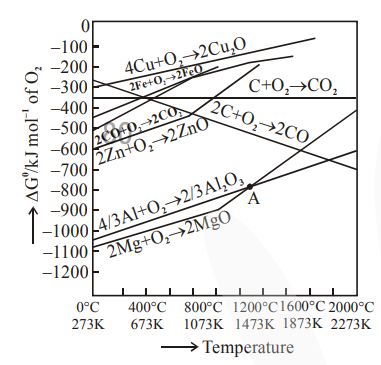# The point of intersection and sudden increase in the slope, in the diagram given below, respectively, indicates:

Question:

The point of intersection and sudden increase in the slope, in the diagram given below, respectively, indicates:1. $\Delta \mathrm{G}=0$ and melting or boiling point of the metal oxide

2. $\Delta \mathrm{G}>0$ and decomposition of the metal oxide

3. $\Delta \mathrm{G}<0$ and decomposition of the metal oxide

4. $\Delta \mathrm{G}=0$ and reduction of the metal oxide

Correct Option: 1

Solution:

At intersection point $\Delta \mathrm{G}=0$ and sudden increase in slope is due to melting or boiling point of the metal.